# Neural Style Transfer

How can you teach a computer to draw like Picasso, Vangogh, Monet or any other artist ? Is it even possible to encode the style of a painting and apply to an existing image ? Gatys et al. introduce a novel concept[^1] that uses Deep neural network to generate artistic images of high perceptual quality.

The paper shows that it is possible to learn the style of a painting (style image) and use it to transform the existing image (content) into an artistic version that appears similar to the painting. In order to make the content image appear artistically similar to the style image, we need a metric that could capture the perceptual style differences between the two.The paper introduces a new approach that uses the deep neural network to extract the content and style features and jointly minimise the white noise image to make its contents similar to content image and style similar to style image. The level of content vs style can be tuned by tuning the amount of content or style to apply.

The paper uses images of painting as reference style image but we can experiment with other style images such as textures, tile maps or even just another photograph.

Proposed network architecture

## Generated Samples

A few samples generated using neural style transfer (Content + Style = Generated)

Content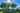Style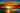Generated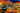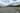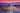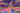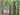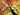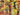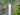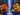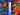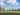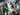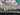## Implementation details

We start with inputs, the content, style and white noise image, run it through the feature extraction process and make use of optimizer to generate the output image.

## Feature extraction

A pretrained convolutional network (vgg19 in the example above) is used to extract the content and style features. For content features, the activation map from a higher layer is used because the higher layers capture objects and their arrangements (the lower layers capture edges and textures). For style features, they use activation maps from multiple layers starting from low to high layers. This helps in generating smoother and visually pleasing images.

## Optimization

The paper uses L-BFGS to minimise the content and style loss by changing white noise image to bring it closer to content and style. We haven’t defined the loss functions yet but we have all the ingredients to define the loss function.

### Content Loss

Minimising the content loss will make white noise image look like source image

$L_{content}(\vec{p},\vec{x},l)=\frac 1 2 \sum_{i,j}(F_{ij}^l - P_{ij}^l)^2$

$\vec{p}$ is content image vector, $\vec{x}$ is white noise image vector and $l$ is the layer

$F_{ij}^l$ is a matrix of feature representation of the content image at layer $l$

$P_{ij}^l$ is a matrix of feature representation of white noise image at layer $l$

$i$ is filter number, $j$ is the position of a filter in the matrix

### Style Loss

The idea of using correlation matrix of feature maps is key to generate an artistic image as it seems to capture the style representation. The paper calls it gram matrix and it is generated by taking inner product between the vectorised feature map. In order to define style loss, we need following:

• gram matrix to capture style representation of each layer
• contribution of each layer to the style loss

Gram matrix

$G^l_{ij}=\sum_k F^l_{ik} F^l_{jk}$

$G_{ij}^l$ is gram matrix at layer $l$

$k$ is the color channel

Layer Loss

The following formula shows the contribution of layer $l$ to the total style loss:

$E_l = \frac{1}{4N^2_lM^2_l} \sum_{i,j}(G^l_{ij} - A^l_{ij})^2$

$E_l$ is the loss at layer $l$

$G_{ij}^l$ is gram matrix from the source image

$A_{ij}^l$ is the gram matrix from white noise image

$N_l$ is a number of feature maps at layer $l$ (# of feature maps = # of filters)

$M_l$ is height x width of feature maps at layer $l$

Style Loss

The style loss is a weighted sum of loss at each layer

$L_{style}(\vec{a}, \vec{x}) = \sum^L_{l=0}w_lE_l$

$\vec{a}$ is source image vector

$\vec{x}$ is white noise image vector

$w_l$ is weighting at layer $l$

Minimising the style loss will make the texture of white noise image look like style image

### Total Loss

We jointly minimise the content and style loss of white noise image using the following equation. $\alpha$ and $\beta$ are the hyperparams that control weighting factors.

$L_{total}(\vec{p},\vec{a}, \vec{x})=\alpha L_{content}(\vec{p}, \vec{x}) + \beta L_{style}(\vec{a}, \vec{x})$

## Conclusion

The paper presents an amazing technique to generate artistic versions of stock photos. The image generation process is slower than other techniques such as real-time style transfer by Johnson et al but allows us to choose any style photo whereas real-time style transfer is done only on pre-trained style images.

References:

1. A Neural Algorithm of Artistic Style [arxiv link] (https://arxiv.org/abs/1508.06576)
2. Justin Johnson’s github repo contains further enhancements such as multiple style images, style interpolation
3. Keras example